# Introduction

With the recent successes in CNNs, the performance of face recognition has also benefited. A baseline for face recognition includes a sequential network and a softmax criterion. In this paper, the authors analyze some disadvantages of the softmax loss, then propose a constraint condition based softmax loss, called L2 softmax loss.

# Problem with softmax loss

Softmax loss has become a standard build-in loss function for a lot of mathematical tools like TensorFlow, Torch and Caffe. It is mainly used for classification, and has its advantages and disadvantages.

1. Separating multiple classes efficiently
2. No restriction on batch selection compared to contrastive or triplet loss
3. Easy implementation

Disadvantages, which this paper mainly focuses on, include:

1. If there are too many classes, it will be problematic for memory fit (a common issue)
2. It fits well to high quality images (see upper figure below) due to its maximization conditional probability. However, it ignores the rare difficult images (see bottom figure below) from the training mini-batch.

The first disadvantage above is thorny unless by using sampled softmax. But for the second one, the author proposes a bound constraint to reduce this negative effect, and result in more attention to the relative hard samples. They call this the L2-softmax constraint, and they use a customized L2 normalization layer and a scaling layer to model this constraint, which we will discuss in the next section.

# L2-Softmax Loss

Since the authors do not want to propose an auxiliary loss like center loss , they directly propose a single sequential network — “one loss system” — which means the L2-softmax loss is a direct alternative for standard sotmax loss, as the equation below:

That’s the overall objective of the L2-softmax loss, where:

• x_i is the input image within a mini-batch of size M
• y_i is the i-th target,
• f(x_i) is the d dimensional feature descriptor before the last fully connected layer
• C is the number of classes
• W and b are the trainable weights and bias in the network

The first equation above is a normal softmax loss formulation, and the second equation above is the constraint equation. Alpha is the most interesting term: it acts as a norm constraint for all feature vectors. A good thing here is it just introduces a single scaling parameter to the network, so it would not influence the overall training speed.

Why add a L2-norm constraint to the network? You may consider it like this: all it does is focuses more on the “bad” samples (with more illumination change, viewpoint change, etc.). Then, the good and bad features would be fixed on a hypersphere, and they would be provided with similar attention due to the L2-norm. An averaged attention to all samples is extremely important for unconstraint environments. When it generalizes well for the poor quality samples, it can be regarded as reducing the relative importance of the very good samples for the softmax classifier. If it comes to a face verification problem, it can strengthen the verification signal by forcing the same class images to be closer and different classes images to be far in the normalized feature space, so the margin between the positive pairs and negative pairs would become larger.

The figure above is an illustration to compare the clustering result between a). Softmax Loss and b). L2-Softmax Loss. Compared to the figure (a), the class variance in figure (b) becomes smaller and the magnitude of the features in figure (b) gets restricted.

As shown in the figure above, the author uses two layers, a L2 Normalization layer and a Scale layer to model this constraint. Basically, this constraint equation below:

is equal to:

which corresponds to what these two layers do (is just to model this constraint equation). The L2 normalize layer normalizes the input feature vector x to a unit vector y. Then the scale layer scales the input unit vector y to a fixed radius given by a parameter alpha. There is only one scalar parameter introduced to the network, which can be trained and also fixed manually.

For the gradient, it should calculate the gradient with respect to the alpha (for trainable alpha) and the gradient with respect to the input x.

The two modules above are fully differentiable, and can be integrated into an end-to-end training network. The following equations are the gradient with respect to alpha and input feature vector x.

# Experiments and Results

The training is based on two different datasets by using L2-softmax loss. One is called MS-small, containing 0.5 million face images with 13403 number of classes. The other is called MS-large, containing 3.7 million face images with 58207 number of classes. The author did lots of comparison experiments between L2-softmax and the standard softmax loss, and also performed evaluations on three popular face verification datasets including IJB-A, LFW and YTF datasets. As the result, the L2-softmax loss outperforms the standard softmax loss.

# Results on IJB-a

The IJB-A (IARPA Janus Benchmark A) dataset includes 5399 still images and 20414 video frames with extreme environments for different viewpoints, resolution and illumination changes.

From the table above, it can be found that regardless of whether alpha acts as a trainable parameter or is fixed manually, the L2 constraint softmax based loss always performs better than softmax loss, especially for small FAR. For example, if we see TAR@FAR = 0.0001 (column 1 in the table), L2-softmax greatly outperforms softmax, which means for some extremely hard samples, L2-softmax did put some attention on them, so that the system learned from such hard samples. Therefore, by given different FAR, the TAR fluctuation of L2-Softmax loss is smaller than the softmax loss.

# Results on LFW

The LFW (Labeled-face in the wild) dataset contains 13233 web-collected frontal face images from 5749 different identities.

As shown in the figure above, for different alpha, when it becomes larger than a threshold (say, 8), the verification accuracy of L2-softmax (red) loss is always better than softmax loss (green), and the best performance of 98.82% accuracy is achieved by using fixed alpha = 16. Note that:

1. the system learned alpha (40.7) is still better than pure softmax (98.02%).

2. if the L2 constraint is too strict, such as fixing alpha to 1 or 4, we can see the accuracy decreases significantly. The reason being that a hypersphere with small radius (alpha) has limited surface area for embedding features from the same class together, and those from different classes far from each other. So the system’s distinguishability is not as good as “a bit more softer“ constraint.

# Results on YTF

YTF (Youtube Face in the Video) dataset contains 3425 videos of 1595 different people, with an average length of 181.3 frames per video. The evaluation protocol settings share same idea with LFW evaluation.

Here, the L2-Softmax achieves 95.54% for YTF dataset evaluation, outperforming softmax (93.82%) again because the L2-softmax puts more attention to the more difficult frames within the videos.

# Some reviewers thoughts

In this paper, the authors add an effective L2-constraint to standard softmax loss for discriminative feature learning. The central idea is to enforce the feature on a fixed radius hypersphere, so that the relative importance of the large magnitude features will become smaller, and small magnitude features become larger, making it possible for the system to pay a balanced attention to all samples, so the rare hard samples will have “more weight”. The overall effect with such a trick results in a big boost to the system’s recognition performance in extreme unconstrained environment. One thing that needs to be improved is the memory fitting problem. Because in this paper, when it comes to MS-large dataset, they use a softmax with 58207 subjects, which really needs to consider the memory fitting problem. Personally, I would like to try some stochastic way to learn a sampled softmax classifier instead.

# Reference

 Y. Wen, K. Zhang, Z. Li, and Y. Qiao. A discrimina- tive feature learning approach for deep face recognition. In European Conference on Computer Vision, pages 499–515. Springer, 2016.

Author: Shawn Yan | Editor: Zhen Gao | Producer: Chain Zhang

--

--

--

## More from SyncedReview

We produce professional, authoritative, and thought-provoking content relating to artificial intelligence, machine intelligence, emerging technologies and industrial insights.

## Accelerating Random Forests Up to 8x Using cuML on Kaggle with Example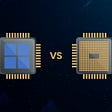## [ Archived Post ] RL Course by David Silver — Lecture 5: Model-Free Control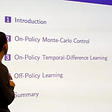## Visualizing Training and Validation Loss in real-time using PyTorch and Bokeh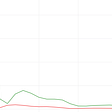## Can graph machine learning identify hate speech in online social networks?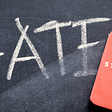## [ Archived Post ] Identifying and attacking the saddle point problem in high-dimensional…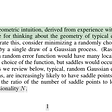## Geometric Deep Learning: Group Equivariant Convolutional Networks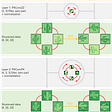## A look under-the-hood of Simply Piano (Part 2)## Benchmarking Machine Learning on the New Raspberry Pi 4, Model B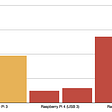## Synced

AI Technology & Industry Review — syncedreview.com | Newsletter: http://bit.ly/2IYL6Y2 | Share My Research http://bit.ly/2TrUPMI | Twitter: @Synced_Global

## VQ-GAN, explained## Diffusion models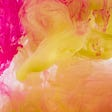## Review: Swin Transformer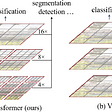## Paper Review: Patch-based inference for TinyML